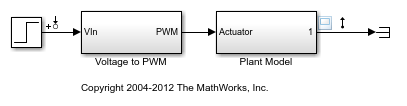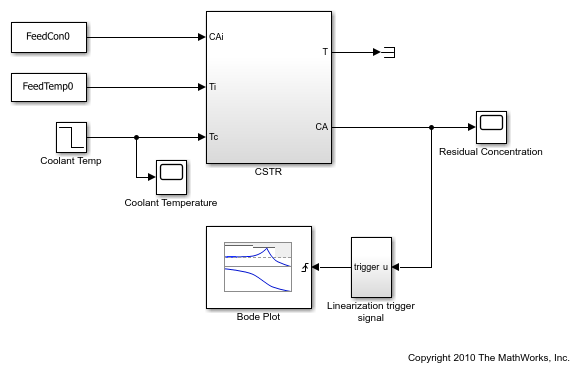Main Content

# getlinio

Obtain linear analysis points from Simulink model, Linear Analysis Plots block, or Model Verification block

## Syntax

``io = getlinio(mdl)``
``io = getlinio(blockpath)``

## Description

example

````io = getlinio(mdl)` returns the analysis points defined in the Simulink® model `mdl`.```

example

````io = getlinio(blockpath)` returns the analysis points defined for the specified Linear Analysis Plots block or Model Verification block in a Simulink model.```

## Examples

collapse all

Open Simulink model.

```mdl = 'scdpwm'; open_system(mdl) ```This model contains the following linear analysis points:

• Input perturbation at the output of the Step block

• Output measurement at the output of the Plant Model block

Obtain the analysis points from the model.

```io = getlinio(mdl) ```
```2x1 vector of Linearization IOs: -------------------------- 1. Linearization input perturbation located at the following signal: - Block: scdpwm/Step - Port: 1 2. Linearization output measurement located at the following signal: - Block: scdpwm/Plant Model - Port: 1 ```

You can use these analysis points for subsequent linearizations of the model using the `linearize` command or an `slLinearizer` interface.

Open Simulink model.

```open_system('scdcstr') ```This model contains a Bode Plot block that is configured with the following linear analysis points:

• Input perturbation at the output of the Coolant Temp block

• Output measurement at the `CA` output of the CSTR block

Obtain the analysis points from the Bode Plot block.

```io = getlinio('scdcstr/Bode Plot') ```
```2x1 vector of Linearization IOs: -------------------------- 1. Linearization input perturbation located at the following signal: - Block: scdcstr/Coolant Temp - Port: 1 2. Linearization output measurement located at the following signal: - Block: scdcstr/CSTR - Port: 2 ```

## Input Arguments

collapse all

Simulink model name, specified as a character vector or string. The model must be in the current working folder or on the MATLAB® path.

If the model is not open or loaded into memory, `getlinio` loads the model into memory.

Linear Analysis Plots block or Model Verification block, specified as a character vector or string that contains its full block path. The model that contains the block must be in the current working folder or on the MATLAB path.

For more information on:

## Output Arguments

collapse all

Analysis point set, returned as a linearization I/O object or a vector of linearization I/O objects. Use `io` to specify linearization inputs, outputs, and loop openings when using the `linearize` command. For more information, see Specify Portion of Model to Linearize.

Each analysis point has the following properties:

PropertyDescription
`Active`

Flag indicating whether to use the analysis point for linearization, specified as one of the following:

• `'on'` — Use the analysis point for linearization. This value is the default option.

• `'off'` — Do not use the analysis point for linearization. Use this option if you have an existing set of analysis points and you want to linearize a model with a subset of these points.

`Block`

Full block path of the block with which the analysis point is associated, specified as a character vector.

`PortNumber`

Output port with which the analysis point is associated, specified as an integer.

`Type`

Analysis point type, specified as one of the following:

• `'input'` — Input perturbation

• `'output'` — Output measurement

• `'loopbreak'` — Loop break

• `'openinput'` — Open-loop input

• `'openoutput'` — Open-loop output

• `'looptransfer'` — Loop transfer

• `'sensitivity'` — Sensitivity

• `'compsensitivity'` — Complementary sensitivity

For more information on analysis point types, see Specify Portion of Model to Linearize.

`BusElement`

Bus element name with which the analysis point is associated, specified as a character vector or `''` if the analysis point is not a bus element.

`Description`

User-specified description of the analysis point, which you can set for convenience, specified as a character vector.

## See Also

### Topics

Introduced before R2006a

## SupportGet trial now